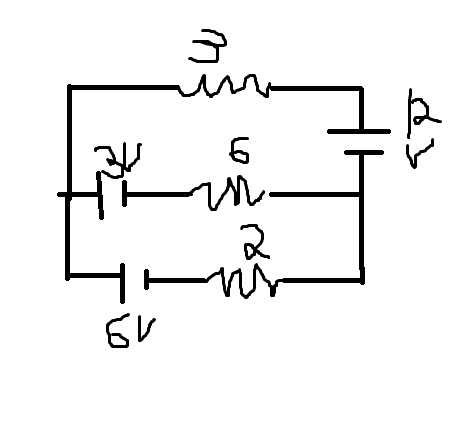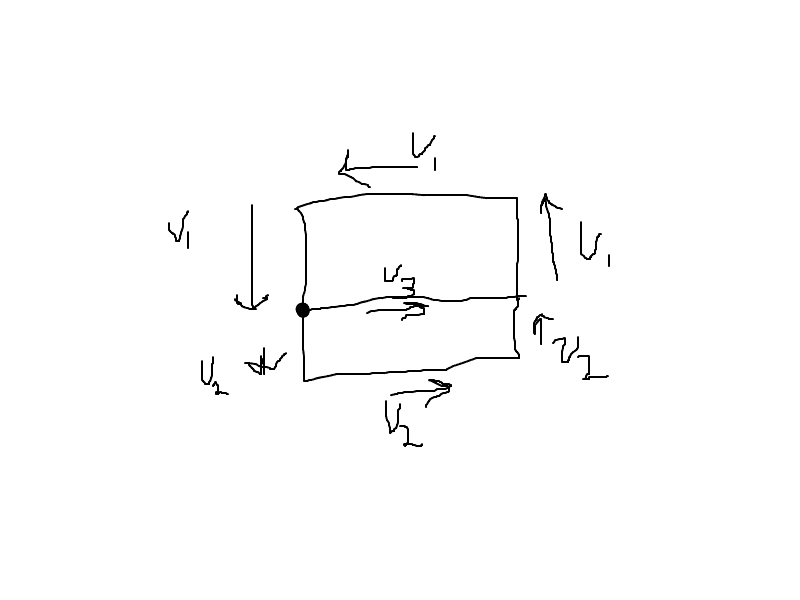# Give magnitude and direction of current flow

## Homework Statement

Problem 3## The Attempt at a Solution

1) Find I and voltage across resistor 5

V=IR
12.6 = I * 5.08
I=2.48

.08*2.48= .1984
12.6-.1984= 12.4 V across battery.

So would I and V across the resistor be I=2.48 and V=12.4?

2) Find current I
(Forgot to mark, I is going clockwise)
Total resistance is 20. Would I simply add the two batteries making V=20?

V=IR 20=I20 I=1?

3) Find current through each resistor. Give magnitude and direction of current flow.

Kind of lost on this one. I have a feeling I need to do kirchoffs rule. How would I handle the battery in the center? I only have examples with the center having a resistor to go off from. The flow was also specified for me. How would I determine the flow if I am not given that information? :(

So far I took the loop counter clockwise from positive end for the top part and current heading towards the right in the center.

12V-3I(1) -6I(2) + 3V= 15V -3I(1)-6I(2) = 0

Bottom counter clockwise from negative end.
-6V-2I(3) +6I(2) -3V = 0
-9V - 2I(3)+6I(2)=0

Look anywhere right so far?

Last edited:

Number one looks OK. Checking the rest now.

For number 2, the total of the batteries voltage is 18V not 20. The total resistance is correct at 20 ohms.

(Note, your batteries would resist each other in this configuration you have drawn giving a total voltage of 6V. I take it that they aren't wired like you have drawn it? Remember, positive is the long leg on the symbol.)

V=IR and you now know V = 18V and R = 20ohms. You can take it from there.

Last edited:

Number one looks OK. Checking the rest now.

For number 2, the total of the batteries voltage is 18V not 20. The total resistance is correct at 20 ohms.

(Note, your batteries would resist each other in this configuration you have drawn giving a total voltage of 6V. I take it that they aren't wired like you have drawn it? Remember, positive is the long leg on the symbol.)

V=IR and you now know V = 18V and R = 20ohms. You can take it from there.

Teehee got the 20 from missadding that.
Yeah I wasn't sure which configuration would result in them opposing or not. I drew the diagram correctly. 6/20 = I

Thanks so much

Teehee got the 20 from missadding that.
Yeah I wasn't sure which configuration would result in them opposing or not. I drew the diagram correctly. 6/20 = I

Thanks so much

So they should be opposing? Then it is 6 not 18 as in my example.

Also, are number 3 supposed to be opposing too? I'll look at 3 once you can confirm this.

yeah it is opposing as well

For circuit 3, the voltage will be equal across all items in the circuit. It is the current through each item which changes.

You will have i1, i2 and i3. Where i1 = i2 + i3.

i1 = Vtotal / R1

and so on for the whole circuit.

You need to analyse this circuit using Kirchoff’s Second Rule (loop rule).

Would the V total be 3?

12 opposed by the 3 and 6 , 12-3-6= 3

V=IR

3=3I
I=1 across top resistor

I(2) + I(3) = 1

Would the I(1)=I(2)+I(3) look something like this?Got pulled away from computer, working on this now. If you happen to see is this, was this what you were trying to say?

Last edited:

In a parallel circuit the voltage is the same across it. So no matter which resistor you measure the voltage across you will read the same value.

It is the current which will vary around the circuit.

In your diagram above, instead of V you should have I, aside from that it is correct.

V1 in your diagram = V2 + V3 But remember, this is current not voltage (again, should be I not V).

Last edited:

So far as voltage goes, I'm not sure how it works in parallel if you have different voltages.

https://www.physicsforums.com/showthread.php?t=67509 shows it isn't a good idea and so far I've found nothing that explains it.

I think you need an electrical engineer to help with this one. Seems a bit complicated for a homework question.

Jared

Yeah I got in a hurry copying from paper to paint and meant the V to be I.

Its the only homework problem from my lab like this and this wasn't even taught to us. *sad face*

This circuit wouldn't work (even without the 12V battery) in real life, it is a pointless exercise.